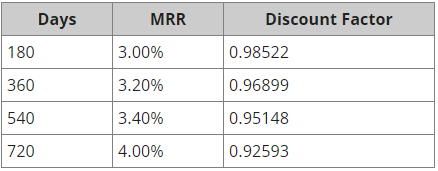# Valuation of Interest Rate Swaps

A $10 million 2-year semi-annual-pay MRR-based interest-rate swap was initiated 180 days ago when swap fixed rate was 3.8%. The fixed rate on the swap is now 3.4% and the term structure is as follows:Value of the swap to the payer is closest to: A) -$45,633
B) -$29,229 C) -$58,114

Correct answer is C. Explanation from Schweser Notes given below.
Sum of the discount factors for the three settlement dates remaining (180,360 and 540 days away) = 0.98522 + 0.96899 + 0.95148 = 2.9057

Value to payer = 2.9057 x [(0.034 – 0.038)/2] x $10 million = -$58,114

I really do not understand this explanation. For some reason I believe this is not the calculation but there is another, simple, proper way of explaining this. Can anybody help? Thank you.

The explanation is correct, but not particularly illuminating.

The value of the new swap is zero (why?), so adding it to your current swap won’t change the total value. If you’re paying the fixed rate on the existing swap, and you receive the fixed rate on the new swap, then the floating rate payments will cancel each other (you’ll receive the floating rate on the old swap and pay it on the new swap, for a net of zero). Therefore, we need to determine only the present value of the fixed payments.

You’ll pay 3.8% of $10 million and receive 3.4% of$10 million in 180 days, and again in 360 days, and again in 540 days. The present values are, respectively,

0.98522\left(\frac{3.4\% - 3.8\%}{2}\right)\$10\ million 0.96899\left(\frac{3.4\% - 3.8\%}{2}\right)\$10\ million

and,

0.95148\left(\frac{3.4\% - 3.8\%}{2}\right)\$10\ million The value is the sum of these, which is: \left(0.98522 + 0.96899 + 0.95148\right)\left(\frac{3.4\% - 3.8\%}{2}\right)\$10\ million
= 2.90569\left(\frac{3.4\% - 3.8\%}{2}\right)\\$10\ million
1 Like

Thank you, but why are we dividing the difference in interest rate by two? Because its semi annual?

Yup.

Interest rates are always – always! – quoted as annual rates.

1 Like

Thank you very much, you really are a magician. I am going to attend my exam on 26th and these things are really helpful. I appreciate what you do.

My pleasure.

Best of luck on your exam, Victor!